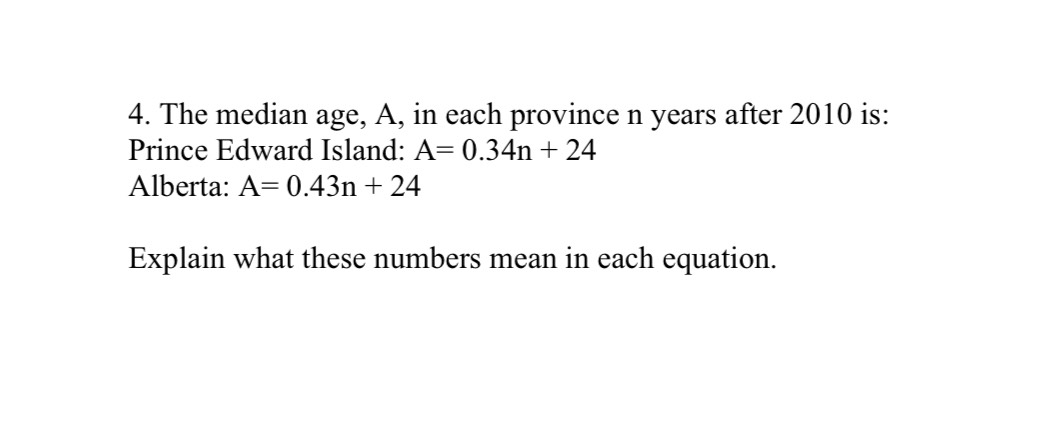### ¿Todavía tienes preguntas de matemáticas?

Pregunte a nuestros tutores expertos
Algebra
Pregunta4. The median age, $$A ,$$ in each province $$n$$ years after $$2010$$ is: Prince Edward Island: $$A = 0.34 n + 24$$ Alberta: $$A = 0.43 n + 24$$ Explain what these numbers mean in each equation.